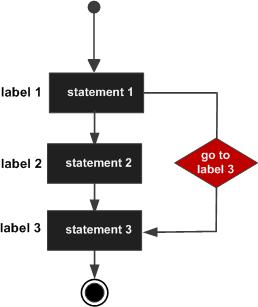# Perl goto Statement

Perl does support a goto statement. There are three forms: goto LABEL, goto EXPR, and goto &NAME.

Sr.No. goto type
1

goto LABEL

The goto LABEL form jumps to the statement labeled with LABEL and resumes execution from there.

2

goto EXPR

The goto EXPR form is just a generalization of goto LABEL. It expects the expression to return a label name and then jumps to that labeled statement.

3

goto &NAME

It substitutes a call to the named subroutine for the currently running subroutine.

## Syntax

The syntax for a goto statements is as follows −

```goto LABEL

or

goto EXPR

or

goto &NAME
```

## Flow Diagram## Example

The following program shows the most frequently used form of goto statement −

```#/usr/local/bin/perl

\$a = 10;

LOOP:do {
if( \$a == 15) {
# skip the iteration.
\$a = \$a + 1;
# use goto LABEL form
goto LOOP;
}
print "Value of a = \$a\n";
\$a = \$a + 1;
} while( \$a < 20 );
```

When the above code is executed, it produces the following result −

```Value of a = 10
Value of a = 11
Value of a = 12
Value of a = 13
Value of a = 14
Value of a = 16
Value of a = 17
Value of a = 18
Value of a = 19
```

Following example shows the usage of goto EXPR form. Here we are using two strings and then concatenating them using string concatenation operator (.). Finally, its forming a label and goto is being used to jump to the label −

```#/usr/local/bin/perl

\$a = 10;
\$str1 = "LO";
\$str2 = "OP";

LOOP:do {
if( \$a == 15) {
# skip the iteration.
\$a = \$a + 1;
# use goto EXPR form
goto \$str1.\$str2;
}
print "Value of a = \$a\n";
\$a = \$a + 1;
} while( \$a < 20 );
```

When the above code is executed, it produces the following result −

```Value of a = 10
Value of a = 11
Value of a = 12
Value of a = 13
Value of a = 14
Value of a = 16
Value of a = 17
Value of a = 18
Value of a = 19
```
perl_loops.htm